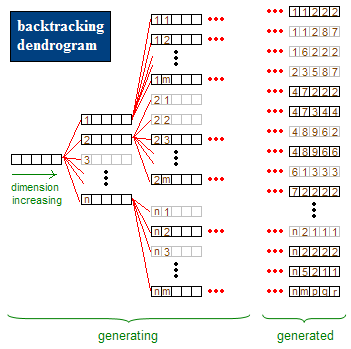2014-01-12

### 源起

1. 我们首先建立一个空栈s,，还有一个输入序列的index，这表示出栈的值，以及即将入栈的元素in，index和in的初始值显然是0,input表示输入的序列；

2. 当in不等于input[index]时，我们将in入栈，in再加1，直到其等于input[index]；

3. in++，index++；这表示4已经顺利出栈；

4. 然后比较s.peek()跟input[index]的值，如果不同，继续循环入栈，相同则出栈；

1. in=0，input=4，将0,1,2,3入栈s；

2. in=4时，in=input,接着in=5,index=1;

3. 栈顶3与序列中input[index]相等，index=2；一直到0都相等；此时，index=5,in=5，栈s为空；

4. 5小于9，将5,6,7,8入栈；

``````public class StackSeq
{
public static boolean isOk(int[] input,int n)
{
int index = 0;
int in = 0;
Stack<Integer> s = new Stack<Integer>();

while(true)
{
if(index >= n - 1)
return true;
if(in >= n)
return false;

if(in != input[index])
{
s.push(in);
++in;
continue;
}

++in;
++index;
while(!s.isEmpty() && s.peek() == input[index])
{
++index;
s.pop();
}

}
}

public static void main(String[] args)
{
StdOut.println("input the number of arrays:");
int[] input = new int[n];

while(true)
{
for (int i = 0 ; i < n ; ++i)
{
}
boolean ret = isOk(input,n);
if(ret == true)
{
StdOut.println("the sequeue is ok!");
}
else
StdOut.println("the sequeue is not ok!");
}

}
}
``````

### 回溯简介``````#include <iostream>
using namespace std;

int count = 0;
void print(int* a,int m)
{
for (int k = 0; k < m; ++k)
{
cout << a[k] << " ";
}
cout << endl;
}

void tuple(int* a,int i,int m,int n)
{

if(i == m)
{
print(a,m);
count++;
return;
}
for (int k = 1; k <= n; ++k)
{
for (int h = 0; h < i; ++h)
{
if(a[h] == k)
{
goto LOOP;
}
}

a[i] = k;
tuple(a,i+1,m,n);
LOOP:
continue;
}
}

int main()
{
int a;
int n,m;
cout << "input C(n,m) :\n";
cin >> n >> m;
cout << "("<< n <<","<< m << ")排列数" << endl;
tuple(a,0,m,n);
}
``````

### 所有可能出栈顺序

``````public static void printiter(int n,int cur,Stack<Integer> tmp,Vector<Integer> out)
``````

n是元素个数，解的维度，cur表示当前的维度，tmp表示2中选择中的进栈，out存放的出栈的元素。终止条件显然是out的元素个数是n。得源码如下：

``````public static void printiter(int n,int cur,Stack<Integer> tmp,Vector<Integer> out)
{
if(n == out.size())
{
for(int i : out)
{
StdOut.print(i + " ");
}
StdOut.println("");
count++;
return;
}

if(cur != n)//入栈
{
tmp.push(cur);
printiter(n,cur + 1,tmp,out);
tmp.pop();
}

if(!tmp.isEmpty())
{
int x = tmp.pop();

printiter(n,cur,tmp,out);
out.remove(out.size() - 1);
tmp.push(x);
}
}
``````

### 八皇后问题

``````bool solution
``````

``````#include <iostream>
using namespace std;

#define N 8

bool solution[N][N] = {false};
void print_solution()
{
for (int i = 0; i < N; ++i)
{
for (int j = 0; j < N; ++j)
{
cout << solution[i][j] <<" ";
}
cout << endl;
}
cout << "\n\n\n";
}

void QueenIter(int x,int y)
{
if(y == N)
{
x++;
y = 0;
}

if(x == N)
{
print_solution();
return;
}

solution[x][y] = true;
QueenIter(x,y+1);

solution[x][y] = false;
QueenIter(x,y+1);
}

int main()
{
QueenIter(0,0);
}
``````

``````void QueenIter(int x,int y,int c)
{
if(y == N)
{
x++;
y = 0;
}

if(x == N)
{
if(c==N)
{
print_solution();
}
return;
}

int d1 = (x+y) % 15;
int d2 = (x-y + 15) % 15;
if(!mx[x] && !my[y] && !md1[d1] && !md2[d2])
{
mx[x] = my[y] = md1[d1] = md2[d2] = true;
solution[x][y] = true;
QueenIter(x,y+1,c+1);
mx[x] = my[y] = md1[d1] = md2[d2] = false;
}

solution[x][y] = false;
QueenIter(x,y+1,c);
}
``````

``````#include <iostream>
using namespace std;

#define N 8
int solution[N] = {0};
bool my,md1,md2;
int count = 0;
void print_solution()
{
for (int i = 0; i < N; ++i)
{
for (int j = 0; j < solution[i]; ++j)
{
cout << 0 << " ";
}
cout << 1 << " ";
for (int j = solution[i] + 1; j < N; ++j)
{
cout << 0 << " ";
}
cout << endl;
}

cout << "\n\n\n";
}

void Queen(int x)
{
if(x == 8)
{
print_solution();
count++;
return;
}

for (int i = 0; i < N; ++i)
{
int d1 = (x+i) % 15;
int d2 = (x-i+15) % 15;
if (!my[i] && !md1[d1] && !md2[d2])
{
my[i] = md1[d1] = md2[d2] = true;
solution[x] = i;
Queen(x+1);
my[i] = md1[d1] = md2[d2] = false;
}

}
}

int main()
{
Queen(0);
cout << count << endl;
}
``````